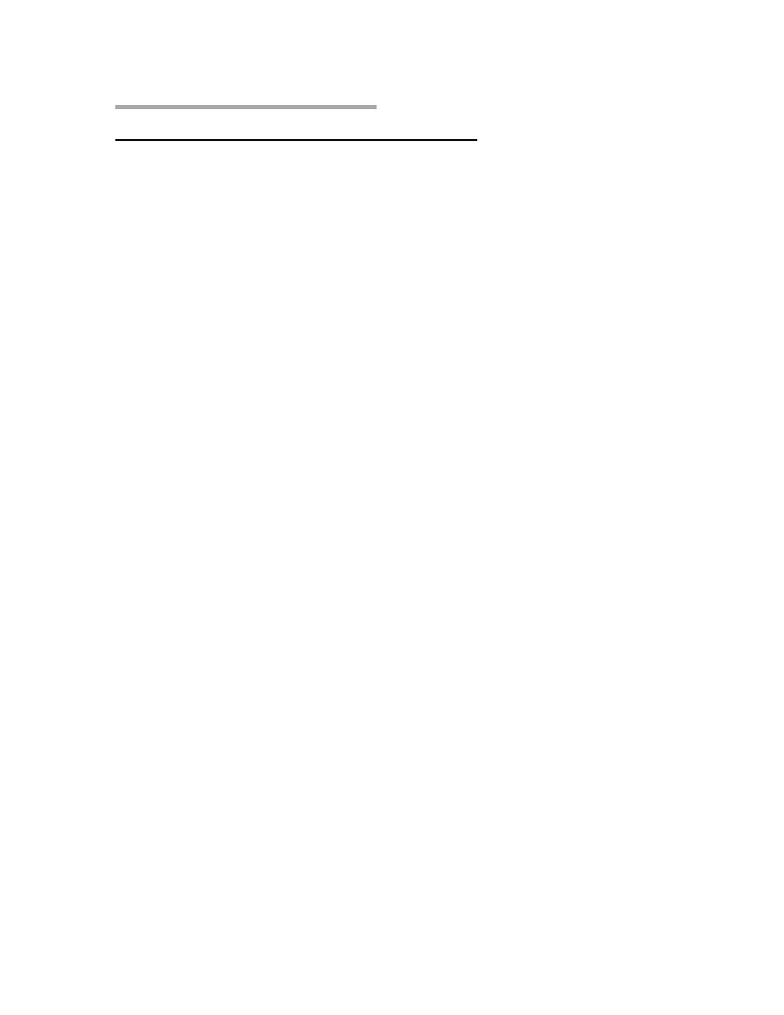# STAB22H3 Chapter Notes - Chapter 4: Quartile, Squared Deviations From The Mean, Interquartile Range

39 views3 pages
School
Department
CourseStats: Data and Models Canadian Edition
Chapter 4 Displaying and Summarizing Quantitative Data
Histograms
- For quantitative variables, there is no obvious way to choose piles so all the possible values are
divided into bins/classes and then the number of cases in each bin/class is counted
- The classes and the counts give the distribution of the quantitative variable
- The histogram displays the distribution at a glance
- Making histograms: aim for 6-10 bins for smaller data sets and 10-25 bins for larger data sets
- Spaces in a histogram are actual gaps in the data (regions where there are no observed values),
whereas in bar graphs, there are spaces between the bars to separate the counts of the different
categories
- Relative frequency histogram replaces the counts with the percentage or proportion of the total
number of cases in each bin/class (shape of histogram will be the same)
Stem-and-Leaf Display
- Like a histogram, but shows the individual values of the data
- Turning the stem-and-leaf on its side should show roughly the same shape as the histogram of the
same data
- The ‘stem’ is the tens digit of the data value (i.e. in 8|4, 8 represents 80)
- The ‘leaf’ is the ones digit of the data value (i.e. 8|4, represents 84)
- With larger data sets, leaves 0-4 and leaves 5-9 are divided to make 2 lines (i.e. with the same
stem)
- 3 digit numbers: the number in the stem can be the hundreds digit, or both the hundreds and the
tens digit together (i.e. 546 can be 5|4 or 54|6), leaves can be two digits, but it is unnecessary
o Leaves are better left as one digit so that there is more room for the data and computers
can better interpret the data
Dotplot
- A dotplot places a dot along an axis for each case in the data
- Like a stem-and-leaf plot, but with dots instead of digits
- Good for small data sets
Think Before you Draw
- Before making a histogram, stem-and-leaf display, or dotplot, check the quantitative data
condition that the data are values of a quantitative variable whose units are known
- Discuss shape, spread, and centre when describing distribution
The Shape of a Distribution
- Humps or peaks in the distribution are called modes
o For categorical variables the mode can be the single value that appears the most often,
but this is not acceptable for quantitative variables
- Histograms can be unimodal, bimodal, or multimodal
- If the histogram can be folded in half (vertically) with the edges matching pretty closely, it is
symmetric
- The thinner ends of the distribution are called tails
- A histogram is skewed to side of the longer tail (if one tail stretches out farther than the other)
- Outliers can be the most informative part of the data or it could be an error don’t just throw it
away; point it out, try to explain it, set it aside, rather than have it distort the data analysis
Unlock document

This preview shows page 1 of the document.
Unlock all 3 pages and 3 million more documents.

# Get access

\$10 USD/m
Billed \$120 USD annually
Homework Help
Class Notes
Textbook Notes